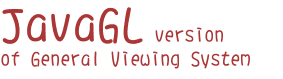Introduction
The General Viewing System (GVS) is used to view the *.tri files, it is developed by using JavaGL.

File Format
There are two modes of the *.tri file format:

Complete Mode:

Triangle
frontcolor_R frontcolor_G frontcolor_B backcolor_R backcolor_G backcolor_B
vertex1_X vertex1_Y vertex1_Z normal1_X normal1_Y normal1_Z
vertex2_X vertex2_Y vertex2_Z normal2_X normal2_Y normal2_Z
vertex3_X vertex3_Y vertex3_Z normal3_X normal3_Y normal3_Z

Simple Mode:

Triangle
vertex1_X vertex1_Y vertex1_Z normal1_X normal1_Y normal1_Z
vertex2_X vertex2_Y vertex2_Z normal2_X normal2_Y normal2_Z
vertex3_X vertex3_Y vertex3_Z normal3_X normal3_Y normal3_Z

Examples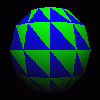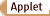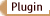Ball Triangles: 120 Size: 25,589 bytes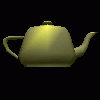Teapot Triangles: 604 Size: 152,784 bytesCSIE dept. building Triangles: 5273 Size: 1,134,121 bytesHuman head Triangles: 2185 Size: 434,916 bytes

Last Updated: Nov. 9 1999